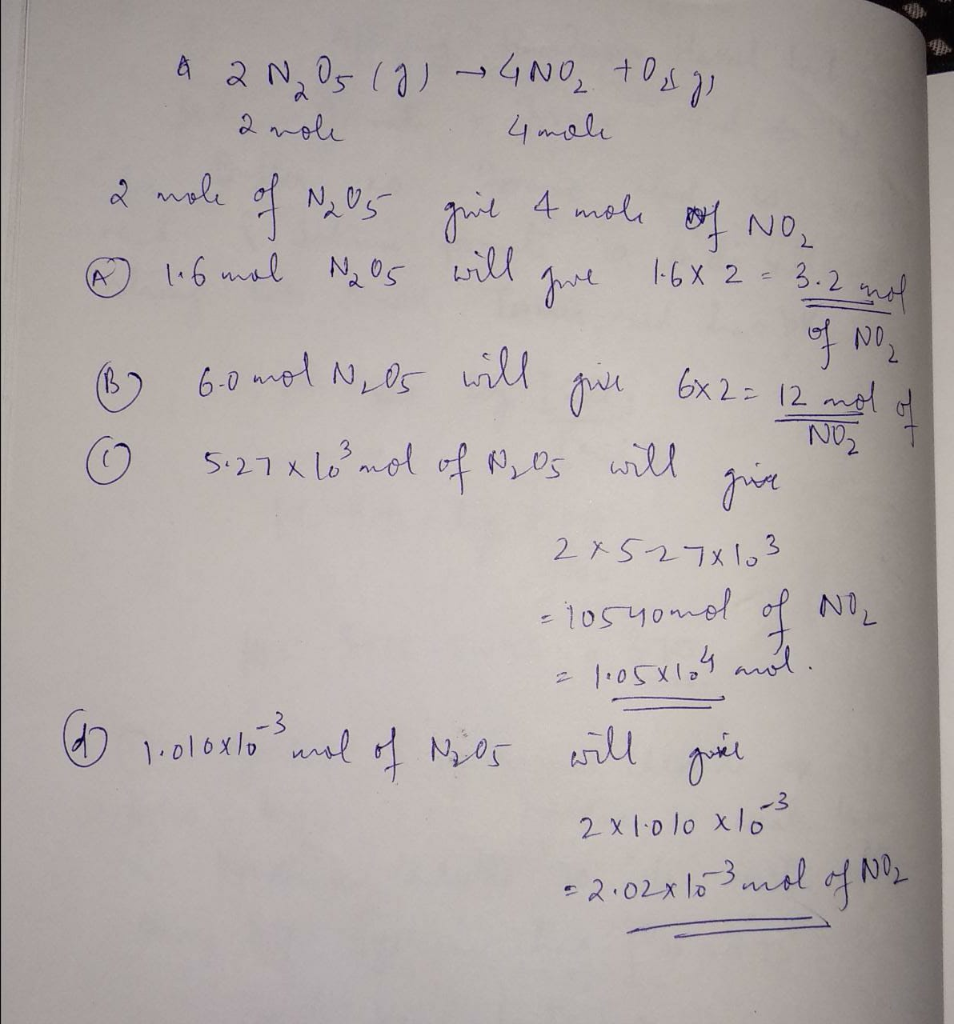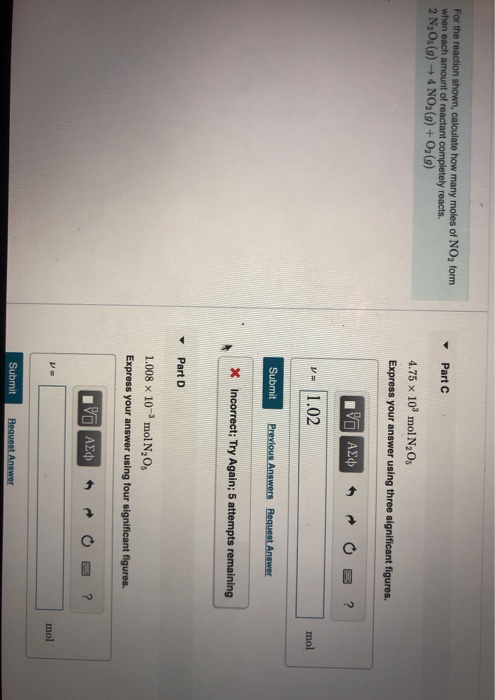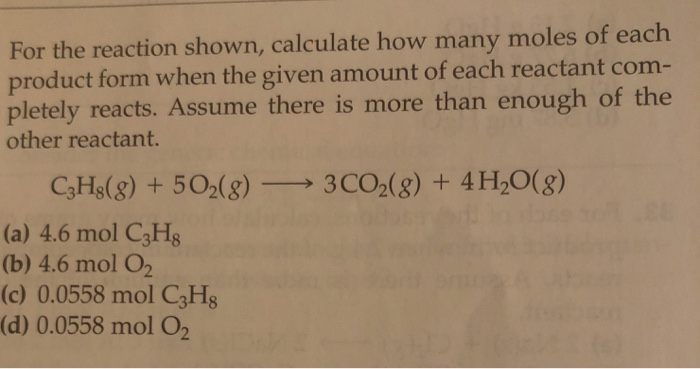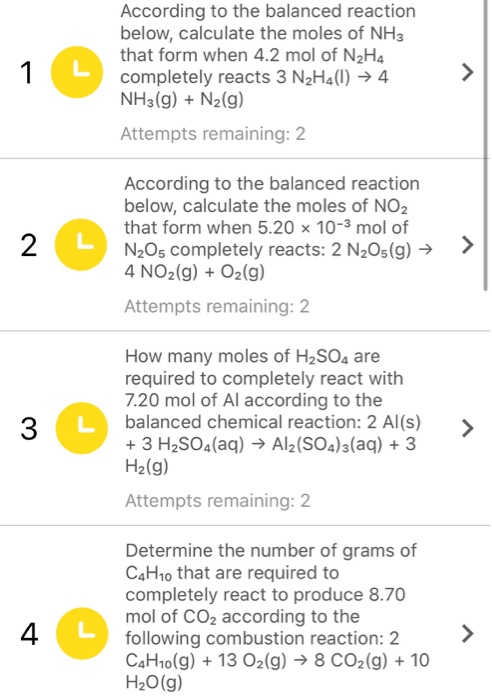Question

For the reaction shown, calculate how many moles of NO2 form when each amount of reactant completely reacts.
2N2O5(g)→4NO2(g)+O2(g)

A. 1.6 mol N2O5

B. 6.0 mol N2O5

C. 5.27×103 mol N2O5

D. 1.010×10−3 mol N2O5#### Earn Coins

Coins can be redeemed for fabulous gifts.

Similar Homework Help Questions
• ### For the reaction shown, calculate how many moles of NO2 form when each amount of reactant...

For the reaction shown, calculate how many moles of NO2 form when each amount of reactant completely reacts. 2N2O5(g)→4NO2(g)+O2(g) Part A 1.4 mol N2O5 Part B   5.6 mol N2O5 Part C   12.5 g N2O5 Part D 1.75 kg N2O5

• ### calculate how many moles of NO2 form when each quantity of reactant completely reacts. 2N2O5(g)--->4NO2(g)+O2(g) a.   2.5...

calculate how many moles of NO2 form when each quantity of reactant completely reacts. 2N2O5(g)--->4NO2(g)+O2(g) a.   2.5 mol N2O5                    b.     6.8 mol  N2O5      c.   15.2 g N2O5                      d.     2.87  kg  N2O5

• ### Calculate how many moles of NO2 form when each quantity of reactant completely reacts. 2N2O5(g)→4NO2(g)+O2(g) 15.8...

Calculate how many moles of NO2 form when each quantity of reactant completely reacts. 2N2O5(g)→4NO2(g)+O2(g) 15.8 g N2O5 2.85 kg N2O5

• ### 2.31 kg k g N2O5 Calculate how many moles of NO2 N O 2 form when...

2.31 kg k g N2O5 Calculate how many moles of NO2 N O 2 form when each quantity of reactant completely reacts via the following reaction: 2N2O5(g)→4NO2(g)+O2(g)

• ### Calculate how many moles of NO2 form when each quantity of reactant completely reacts. 2N2O5(g)→4NO2(g)+O2(g) 1....

Calculate how many moles of NO2 form when each quantity of reactant completely reacts. 2N2O5(g)→4NO2(g)+O2(g) 1. 2.9 mol N2O5 Express your answer using two significant figures. 2. 7.0 mol N2O5 Express your answer using two significant figures. 3.    16.6 g N2O5 Express your answer using three significant figures. 4.    2.39 kg N2O5 Express your answer using three significant figures.

• ### for the reaction shown. calculate how many moles of no2 form when each of the following...

for the reaction shown. calculate how many moles of no2 form when each of the following completely reacted.2 N2O5(g)---->4NO2(g) + O2(g)

• ### For the following reaction, calculate how many moles of NO2, forms when 0.356 moles of the...

For the following reaction, calculate how many moles of NO2, forms when 0.356 moles of the reactant completely reacts. 2N2O5(g) yields 4NO2(g)+ O2(g)

• ### For the reaction shown, calculate how many moles of NO, form when each amount of reactant...For the reaction shown, calculate how many moles of NO, form when each amount of reactant completely reacts. 2 N,Os(9) + 4NO2(g) + O2(9) Part C 4.75 x 10' mol N205 Express your answer using three significant figures. AXO O ? % 1.02 mol Submit Previous Answers Request Answer * Incorrect; Try Again; 5 attempts remaining Part D 1.008 x 10-mol N, O, Express your answer using four significant figures. V ASD - O ? mol Submit Request Answer

• ### For the reaction shown, calculate how many moles of each product form when the given amount...For the reaction shown, calculate how many moles of each product form when the given amount of each reactant com- pletely reacts. Assume there is more than enough of the other reactant. C3H2(g) + 5O2(g) — 3CO2(g) + 4H2O(8) (a) 4.6 mol C3H (b) 4.6 mol O2 (c) 0.0558 mol C3H (d) 0.0558 mol O2

• ### According to the balanced reaction below, calculate the moles of NH3 that form when 4.2 mol of N2H4 completely reac...According to the balanced reaction below, calculate the moles of NH3 that form when 4.2 mol of N2H4 completely reacts 3 N2H4(1) ► 4 NH3(g) + N2(g) Attempts remaining: 2 According to the balanced reaction below, calculate the moles of NO2 that form when 5.20 x 10-3 mol of N205 completely reacts: 2 N2O5(g) → 4 NO2(g) + O2(g) Attempts remaining: 2 How many moles of H2SO4 are required to completely react with 7.20 mol of Al according to the...